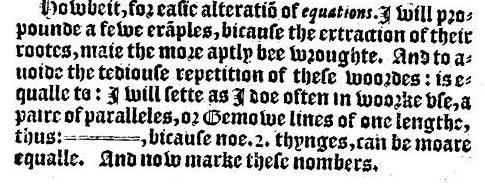Search IntMath
Close

# The signs used in arithmetic

By Murray Bourne, 08 Aug 2011

Reader Lemmie sent me an interesting excerpt from a book The Children's Treasure House Vol 5 How and Why, by Arthur Mee, printed in 1926.

It contains an interesting passage about the origin of signs used in arithmetic.

Lemmie is a "mature" reader and said "This is from an old set of books in the bookshelf of my late mum. It was my 'TV' back in the late 40s".

I've mostly quoted directly from the excerpt, but added extra information in square brackets and some links to more information. Some of the notation explanations are somewhat speculative, I suspect.

## THE SIGNS USED IN ARITHMETIC

The signs that we use in arithmetic are known to all, but their origin is not so familiar.

The sign =, meaning equal to, was first used by [Welshman] Robert Recorde, of All Souls' College, Oxford, in 1531. To save himself the trouble of writing the words "equal to" again and again, he drew two little lines equal to one another. [He said "Noe 2 thynges can be moare equalle” than parallel lines. His symbol appears to be 5 times the current length of the equal sign, as we can see in The Whetstone of Witte by Recorde, below.]Image source with text

The sign for addition (+) is really a carelessly made p, from plus, the Latin word for more.

The —, for subtraction, also comes from a shortened Latin word, minus, meaning "less than", which was written m n s, with a horizontal stroke on top to show that it had been shortened. Then the letters were omitted, and the stroke only written.

The multiplication sign (×) was invented early in the seventeenth century by Oughtred Etonensis, the most famous mathematician then in Europe [aka William Oughtred, who contributed to inventing the slide rule, and gave us "sin" and "cos" in trigonometry]. It was simply the + sign turned round, multiplication being a short way of doing addition. [There is some debate about this notion. See Devlin's Angle where he argues It Ain't No Repeated Addition - unfortunately this is no longer available.]

In division the Hindus used to put the dividend [the number you divide into] above the divisor [the number you are dividing by] with a horizontal line between, and from this plan the Arabians developed the sign ÷, placing it between the dividend and divisor. [This "divide" sign is often hard to read, since it can look a lot like a plus sign, depending on the font used and font size.]

The sign % for per cent has developed from ÷, once used for per cent as well as for division.

The radical sign (√), meaning that the square root of a number is to be taken, is really the first letter (r), of the Latin word radix, meaning root. [The radical sign was introduced by Christoff Rudolff (Polish, 1499 - 1545).]

The • (dot) as used in decimal fractions was invented by John Napier [1550 - 1617], the man who also invented logarithms.

The n used in algebra to signify any indefinite number is the initial letter of the Latin word numerus, meaning a number.

## OTHER SIGNS [also used in math]

The present question mark (?) and the exclamation mark (!) have a similar and an interesting origin.

The ! (exclamation mark) represents the Latin exclamation lo, which was used to signify a cry of joy. When the Latin writers wished to signify joy they wrote this word, then, so that it might not be read as a part of the verse or line, they wrote the letters one above the other, thus 10, and this, in rapid writing, soon developed into "!".

[Interestingly, you often hear in Asia the word "aiyoh" to mean surprise or frustration. This could be linguistically related to the Latin Io.]

The ? (question mark) came similarly from the first and last letters of the Latin word questio, meaning question, written one above the other in the same way Q0. The Q written quickly became [the top of the question mark symbol] and the o became a point.

## Concluding remark

Much of our math notation is relatively quite recent and was introduced as Europe emerged from the Dark Ages. We act as though the notation is fixed an unchangeable, but I think we are overdue for math notation reform. See: Toward more meaningful math notation.

### Comment Preview

HTML: You can use simple tags like <b>, <a href="...">, etc.

To enter math, you can can either:

1. Use simple calculator-like input in the following format (surround your math in backticks, or qq on tablet or phone):
a^2 = sqrt(b^2 + c^2)
(See more on ASCIIMath syntax); or
2. Use simple LaTeX in the following format. Surround your math with $$ and $$.
$$\int g dx = \sqrt{\frac{a}{b}}$$
(This is standard simple LaTeX.)

NOTE: You can mix both types of math entry in your comment.